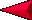Applied Science - Science and Math (3B) Post Lab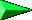OBJECTIVES: Comparing volume, mass, and weight. Investigating different methods of measuring. VOCABULARY: mass volume weight MATERIALS: balance (bucket) spring balance liter boxes bath scale graduated cylinders Students look at instruments that measures mass and weight.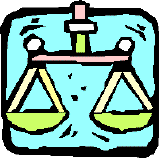BACKGROUND: Mass is the quantity of matter in a body.  More specifically, it is the measurement of the inertia or sluggishness that a body, in the absence of friction, exhibits in response to any effort made to start it, stop it, or change in any way from its original state of motion.  Two items that have the same dimensions could have different masses, and two different sized objects could have similar mass.  Mass does not change, no matter if you are in space or on Earth.  Mass is a measure of the matter within a body.  Mass is measured in kilograms and grams (metric) and slugs (English). Weight is the force of gravitational attraction on a body as observed on the rotating Earth or similar situation.  Weight on Earth and weight on the Moon of the same object, is different.  The English system is measured in ounces and pounds and the metric is measured in newtons and dynes.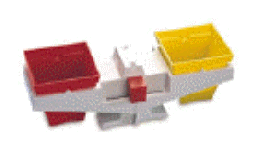Although the difference between mass and weight is real, on Earth mass and weight are very similar.  Many books do not distinguish the difference.  This activity introduces the two words, mass and weight, and acknowledges they are somehow different.  This helps students in future labs. Volume is the quantity of space a body occupies measured in liters and milliliters in the metric system and ounces and pounds in the English system.  The same volume of two different substances, may have different weight and mass. Students in the third grade just have to understand that weight and mass are similar but not the same.  Mathematical formulas can be used to distinguish the two easier, but not until a later grade!  PROCEDURE: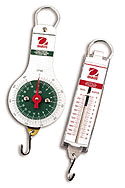Go over the difference between volume, mass, and weight.  Emphasize that on Earth the two measurements are similar, but in space the differences are very important.  For instance you will weigh much less on the Moon than on Earth, but your mass is still the same.   Gravity influences weigh, so the larger the body like Jupiter, the more you will weigh.  It is not important if children remember this, as much as you begin their understanding of the difference.     If you have different scales and balances you should demonstrate mass, volume and weight.  A balance measures mass. A bath scale measures weight.  Liter boxes or graduated cylinders measure volume.  Go over this several times.  It is not easy to understand, but very important in later labs.  Have the students measure different things on the balance so they become familiar with using the balance.    You may want to use the worksheet and have students identify which of the instruments are used to measure volume, weight, and mass.  The graduated cylinder measures volume, the rest of the scales (spring, balance, and scale) are used to measure weight and mass depending on the type of scale used.